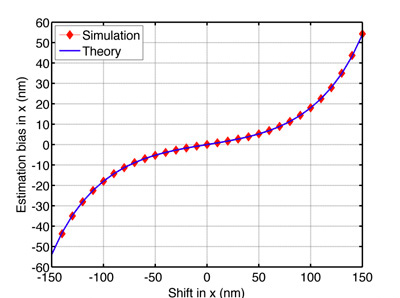Dr. Zhaolong Shen and Dr. Sean B. Andersson (PI), Mechanical Engineering, Boston University

### IntroductionThe objective of this project is to investigate the performance of a point source position estimator. By taking advantage of parallel processing on SCV’s Katana Cluster, the researchers were able to generate the fluorescent intensity distribution of a point source with nano-meter accuracy. The estimation bias and variance of the estimator were then simulated by applying the generated data and compared to the theoretical predictions.

This research was funded by NSF grants DBI-0649823 and CMMI-0845742.

### RCS Contribution

The numerical simulations were initially carried out on an Apple Mac using MATLAB. Some calculations took as much as 30 days to complete which was an impediment for moving the project forward because of the long runtime. Kadin Tseng of RCS was solicited for help to speed up the computational process. While compiled languages such as C or Fortran coupled with MPI may be used, this particular case had many other considerations which dictated that MATLAB should be looked at first for performance improvement. With a handful of performance enhancing MATLAB programming techniques (such as array preallocation and vectorization of complex arithmetic expressions to eliminate nested loops) along with the use of MATLAB’s parallel-processing enabled ODE functions, the runtime was reduced to approximately 7 hours. With this greatly reduced runtime, many more numerical simulations may be — and were — pursued without a long wait time.